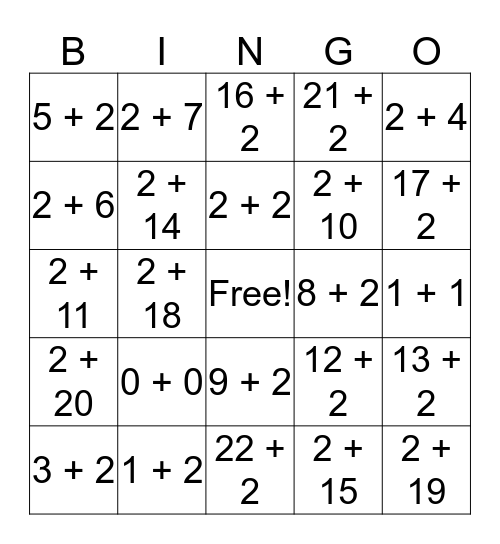This bingo card has a free space and 24 words: 0 + 0, 1 + 2, 2 + 2, 3 + 2, 2 + 4, 5 + 2, 2 + 6, 2 + 7, 8 + 2, 9 + 2, 2 + 10, 2 + 11, 12 + 2, 13 + 2, 2 + 14, 2 + 15, 1 + 1, 16 + 2, 17 + 2, 2 + 18, 2 + 19, 2 + 20, 21 + 2 and 22 + 2.

## Play Online